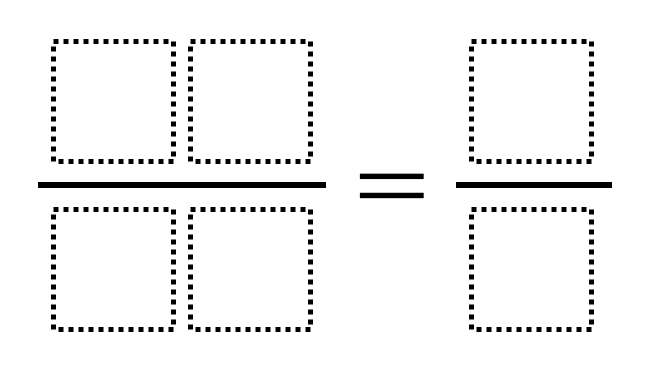# Unit Rate

Directions: Using the digits 0 to 9 at most one time each, place a digit in each box to make two equivalent ratios where one of the ratios is a unit rate.### Hint

1. What do we know about a unit rate?
2. Are any numbers off-limits in this problem?

There are many answers including 68/34 = 2/1 and 78/26 = 3/1. All of them will have a 1 in the denominator of the fraction on the right.

Source: Scott Houston

## Greatest Common Factor

Directions: Using the digits 0 to 9 at most one time each, place a digit …

1.Diana Khutsaeva

72/24 =3/1

2.86/43 = 2/1
70/35 = 2/1
90/45 = 2/1
68/34 = 2/1

3.4.5.•I defied Open middle with my answer

•